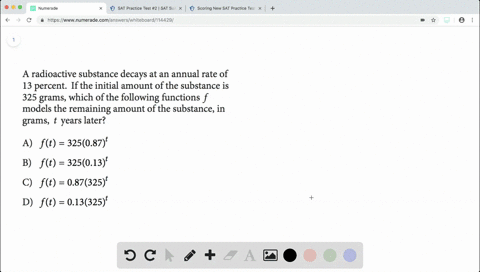Enroll in one of our FREE online STEM summer camps. Space is limited so join now!View Summer Courses### A radioactive substance decays at an annual rate …

01:19Johns Hopkins University

Need more help? Fill out this quick form to get professional live tutoring.

Get live tutoring
Problem 13

The function $h,$ defined by $h(t)=a t+b,$ where $a$
and $b$ are constants, models the height, in
centimeters, of the sunflower after $t$ days of growth
during a time period in which the growth is
approximately linear. What does a represent?
A) The predicted number of centimeters the
sunflower grows each day during the period
B) The predicted height, in centimeters, of the
sunflower at the beginning of the period
C) The predicted height, in centimeters, of the
sunflower at the end of the period
D) The predicted total increase in the height of the
sunflower, in centimeters, during the period

Check back soon!
SAT
SAT Practice Test # 6

## Discussion

You must be signed in to discuss.

## Video Transcript

so again, it says the function H is defined by age of tea is he could eighty plus B where A and B are Constance. Well, that looks like a form of Isaac. What's a next class B, where M is our slope? So they've just replaced with A and X with tea. That's the same thing. So we know that em and they are the same thing. Well, that means that M and A are both slopes, which are rates. So we're looking for something that talks about, like rats either a slope or a rate or something like that. So let's look at it So A says the predictive number of centimeters to sun flower goes each day during the period. Well, so they're talking about growth per day, so that sounds like a rate rose per day. So that's probably good answer. But let's go in and go through the other ones, he says. The heightened centimeters of the something at the very beginning. Well, that zero, and that's the white intercepts. So we know that that one is actually that be value, so that's not correct, See, says predicted, heightened centimeters at some flower at the end of period. Well, that's just this value. And that's just a value of age of eighty four. Because that's at the very advanced. Let's not sea bass is the predicted total increase in the height of the sunflower and centimeters during the period. Well, the total predicted height increase is just the difference between the final value and the initial value. So that's also not a rate we don't want to choose that, so a is going to be our correct answer.